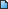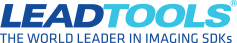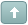Error

# HOW TO: Determine if a PDF is Searchable in V20

#1 Posted : Friday, December 22, 2017 1:56:51 PM(UTC)
Below you will find the updated code for our demo application used to determine if a PDF is Searchable.

These first two examples (C# and VB) test the first page of the PDF to see if text is available to be read.
determinePdfSearchable_20
determinePdfSearchableVB_20

The second two examples (C# and VB) provide an series of checks to run against the PDF to see if it is valid in a number of ways.
pdfValidation_20
pdfValidationVB_20

C#
Code:
``````
string licFile = @"";
string keyFile = "";

string result = "This PDF is NOT Readable";

PDFDocument pdfDoc = new PDFDocument(pdfFile);
pdfDoc.ParsePages(PDFParsePagesOptions.AllIgnoreWhiteSpaces, 1, 1); // For this sample we only check the first page
PDFDocumentPage firstPage = pdfDoc.Pages;

if(firstPage.Objects.ObjectType == PDFObjectType.Text) // Indicates the document has searchable text
{
result = "Yes, This PDF is Readable";
Console.WriteLine(result);
}

VB
Code:
``````
Sub Main()
Dim keyFile As String = ""

Dim pdfFile As String = "C:\Users\cthompson\Desktop\leadtools.pdf"
Dim result As String = "This PDF is NOT Readable"

Dim pdfDoc As PDFDocument = New PDFDocument(pdfFile)
pdfDoc.ParsePages(PDFParsePagesOptions.AllIgnoreWhiteSpaces, 1, 1) 'For this sample we only check the first page

Dim firstPage As PDFDocumentPage = pdfDoc.Pages(0)

If firstPage.Objects(0).ObjectType = PDFObjectType.Text Then
result = "Yes, This PDF is Readable"
Console.WriteLine(result)
End If

End Sub``````

Code for PDF Validation

C#
Code:
``````
static void Main(string[] args)
{
string licFile = @"";
string keyFile = "";
RasterCodecs codecs = new RasterCodecs();

string[] data = { "Filename:   ", "Readable?   ", "Is a PDF?   ", "Encrypted?  ", "Searchable? " };

data += Path.GetFileName(pdfFile);

try
{
CodecsImageInfo fileInfo = codecs.GetInformation(pdfFile, false);  // Determine if file is valid (if the file is corrupted this will throw an exception)
data += "Yes";

if (IsPDF(fileInfo.Format))   // Some corrupted files do not throw an exception, but we cannot determine their format.  So check to ensure we see the file as a PDF.
// Also, some files may just have the PDF extension, but are not actually PDF files.  It is best to ensure we see it as a PDF file.
{
data += "Yes";
if (!PDFFile.IsEncrypted(pdfFile))  // Determine if the file requires a password to open
{
data += "No";
PDFDocument pdfDoc = new PDFDocument(pdfFile); // Create PDFDocument object to determine if file is searchable or not
pdfDoc.ParsePages(PDFParsePagesOptions.AllIgnoreWhiteSpaces, 1, 1); // For this sample we only check the first page
PDFDocumentPage firstPage = pdfDoc.Pages;
for (int i = 0; i < firstPage.Objects.Count; i++)
{
if (firstPage.Objects[i].ObjectType == PDFObjectType.Text) // Indicates the document has searchable text
{
data += "Yes";
break;
}
}
}
else
{
data += "Yes";
data += "N/A";
}
}
else
{
data += "No";
data += "N/A";
data += "N/A";
}
}
catch (Exception ex)
{
// If we throw "PDF Error - File is corrupted" we've determined the file to be PDF, but it is corrupted and cannot be read
if (ex.Message == "PDF Error - File is corrupted")
{
data += "No";
data += "Yes";
data += "N/A";
data += "N/A";
}
}

DisplayResults(data);
}

private static void DisplayResults(string[] data)
{
foreach (string result in data)
{
Console.WriteLine(result);
}
}

private static bool IsPDF(RasterImageFormat rasterImageFormat)
{
return ((rasterImageFormat == RasterImageFormat.RasPdf) ||
(rasterImageFormat == RasterImageFormat.RasPdfCmyk) ||
(rasterImageFormat == RasterImageFormat.RasPdfG31Dim) ||
(rasterImageFormat == RasterImageFormat.RasPdfG32Dim) ||
(rasterImageFormat == RasterImageFormat.RasPdfG4) ||
(rasterImageFormat == RasterImageFormat.RasPdfJbig2) ||
(rasterImageFormat == RasterImageFormat.RasPdfJpeg) ||
(rasterImageFormat == RasterImageFormat.RasPdfJpeg411) ||
(rasterImageFormat == RasterImageFormat.RasPdfJpeg422) ||
(rasterImageFormat == RasterImageFormat.RasPdfLzw) ||
(rasterImageFormat == RasterImageFormat.RasPdfLzwCmyk));
}``````

VB
Code:
``````Sub Main()
Dim licFile As String = ""
Dim keyFile As String = ""
Dim codecs As RasterCodecs = New RasterCodecs()
Dim pdfFile As String = "C:\Users\cthompson\Desktop\leadtools.pdf"

Dim Data As String() = {"Filename:   ", "Readable?   ", "Is a PDF?   ", "Encrypted?  ", "Searchable? "}

Data(0) += Path.GetFileName(pdfFile)

Try

Dim fileInfo As CodecsImageInfo = codecs.GetInformation(pdfFile, False)  ' Determine if file Is valid (if the file Is corrupted this will throw an exception)
data(1) += "Yes"

' Some Then corrupted files Do Not Throw an exception, but we cannot determine their format.  So check To ensure we see the file As a PDF.
' Also, some files may just have the PDF extension, but are Not actually PDF files.  It Is best to ensure we see it as a PDF file.
If IsPDF(fileInfo.Format) Then

data(2) += "Yes"

If Pdf.PDFFile.IsEncrypted(pdfFile) = False Then ' Determine Then If the file requires a password To open

Data(3) += "No"
Dim pdfDoc As PDFDocument = New PDFDocument(pdfFile) ' Create PDFDocument object to determine if file Is searchable Or Not
pdfDoc.ParsePages(PDFParsePagesOptions.AllIgnoreWhiteSpaces, 1, 1) ' For this sample we only check the first page
Dim firstPage As PDFDocumentPage = pdfDoc.Pages(0)
For i As Integer = 0 To firstPage.Objects.Count '(Int() i = 0; i < firstPage.Objects.Count; i++)

If (firstPage.Objects(i).ObjectType = PDFObjectType.Text) Then ' Indicates the document has searchable text

Data(4) += "Yes"
Exit For

End If
Next

Else
Data(3) += "Yes"
data(4) += "N/A"
End If
Else
data(2) += "No"
data(3) += "N/A"
data(4) += "N/A"
End If

Catch ex As Exception
' If we throw "PDF Error - File is corrupted" we've determined the file to be PDF, but it is corrupted and cannot be read
If ex.Message = "PDF Error - File is corrupted" Then

data(1) += "No"
data(2) += "Yes"
data(3) += "N/A"
data(4) += "N/A"

End If
End Try

DisplayResults(data)

End Sub
Sub DisplayResults(ByRef data As String())
For Each result As String In data
Console.WriteLine(result)
Next

End Sub
Function IsPDF(ByRef rasterImageFormat As RasterImageFormat) As Boolean
Return (rasterImageFormat = RasterImageFormat.RasPdf) Or
(rasterImageFormat = RasterImageFormat.RasPdfCmyk) Or
(rasterImageFormat = RasterImageFormat.RasPdfG31Dim) Or
(rasterImageFormat = RasterImageFormat.RasPdfG32Dim) Or
(rasterImageFormat = RasterImageFormat.RasPdfG4) Or
(rasterImageFormat = RasterImageFormat.RasPdfJbig2) Or
(rasterImageFormat = RasterImageFormat.RasPdfJpeg) Or
(rasterImageFormat = RasterImageFormat.RasPdfJpeg411) Or
(rasterImageFormat = RasterImageFormat.RasPdfJpeg422) Or
(rasterImageFormat = RasterImageFormat.RasPdfLzw) Or
(rasterImageFormat = RasterImageFormat.RasPdfLzwCmyk)
End Function
``````

File Attachment(s):determinePdfSearchable_20.zip (32kb) downloaded 125 time(s).

File Attachment(s):determinePdfSearchableVB_20.zip (52kb) downloaded 104 time(s).

File Attachment(s):pdfValidation_20.zip (34kb) downloaded 99 time(s).

File Attachment(s):pdfValidationVB_20.zip (53kb) downloaded 90 time(s).
Chris Thompson
Developer Support EngineerTry the latest version of LEADTOOLS for free for 60 days by downloading the evaluation: https://www.leadtools.com/downloadsWanna join the discussion? Login to your LEADTOOLS Support accountor Register a new forum account.